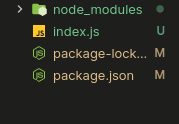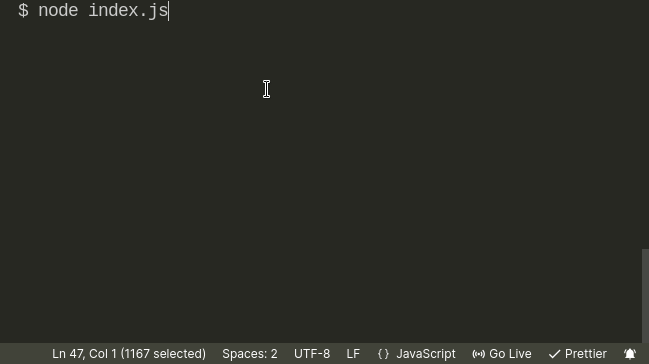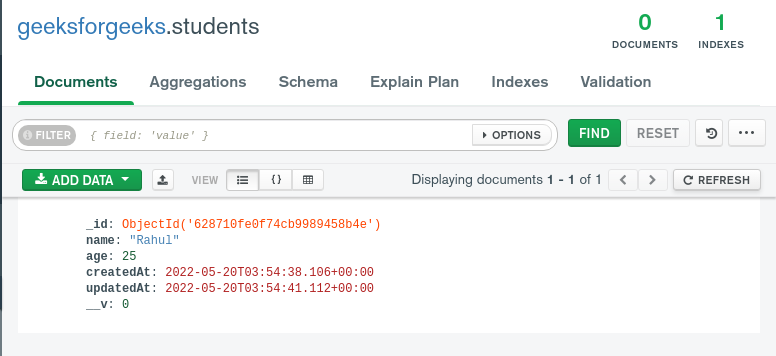# Mongoose Timestamps

• Last Updated : 25 May, 2022

Mongoose is a MongoDB object modelling and handling for node.js environment.

Mongoose timestamps are supported by the schema. Timestamps save the current time of the document created and also when it was updated in form of a Date by turning it true. When set to true, the mongoose creates two fields as follows:

• createdAt: Date representing when the document was created
• updatedAt: Date representing when this document was last updated

The two fields are created when the database was first created and then updated by the queries save(), updateOne(), updateMany(), findOneAndUpdate(), update(), replaceOne(), or bulkWrite().

Syntax: Create schema with a timestamp as follows:

const studentSchema = new Schema({ name: String }, { timestamps: true });

const Student = mongoose.model(‘Student’, studentSchema);

Creating Application and installing Modules: We will create a schema with the timestamps and then print the different timestamps that are createdAt and updatedAt by updating the details of a student.

Step 1: Create a folder and initialize it:

`npm init`

Step 2: Install mongoose in the project.

`npm i mongoose`

Project Structure: The project structure is as follows:Example: Create a file called index.js. Inside the index.js, connect to MongoDB. Here the MongoDB Compass is used. Now first create the Student schema and then its model. Now create a new document and save it. Print the document timestamps and then update the document after a time delay and then again print the timestamp details.

## index.js

 `const mongoose = require(``"mongoose"``); ` ` `  `// Database connection ` `mongoose.connect(``"mongodb://localhost:27017/geeksforgeeks"``); ` ` `  `// Creating Schema ` `const studentSchema = ``new` `mongoose.Schema({ ` `        ``name: { type: String, required: ``true` `}, ` `        ``age: { type: Number, ``default``: 8 }, ` `    ``}, ` `    ``{ timestamps: ``true` `} ` `); ` ` `  `// Student model ` `const Student = mongoose.model(``"Student"``, studentSchema); ` ` `  `// Creating Student document from Model ` ` `  `// Function to save in database ` `const saveStudent = async (name, age) => { ` `    ``let s = ``new` `Student({ ` `        ``name: name, ` `        ``age: age, ` `    ``}); ` `    ``s.save().then((doc) => { ` `        ``console.log(``"Name:"``, doc.name, ``", Age:"``, doc.age); ` `        ``console.log(``"Created At:"``, doc.createdAt); ` `        ``console.log(``"Updated At:"``, doc.updatedAt); ` `    ``}); ` `}; ` ` `  `const updateStudent = async () => { ` `    ``let doc = await Student.findOneAndUpdate( ` `        ``{ name: ``"Rahul"` `}, ` `        ``{ age: 25 }, ` `        ``{ ``new``: ``true` `} ` `    ``); ` `    ``console.log(``"Name:"``, doc.name, ``", Age:"``, doc.age); ` `    ``console.log(``"Created At:"``, doc.createdAt); ` `    ``console.log(``"Updated At:"``, doc.updatedAt); ` `}; ` ` `  `const start = async () => { ` `    ``await saveStudent(``"Rahul"``, 15); ` `    ``setTimeout(``function` `() { ` `        ``updateStudent(); ` `    ``}, 3000); ` `}; ` ` `  `start(); `

Step 4: Run the code using the following command:

`node index.js`

Output: The output in the command line is as follows. The output shows that the document is updated after 3 seconds.Output of MongoDB: The following field will now reflect in the database also, as shown in the image below:My Personal Notes arrow_drop_up
Recommended Articles
Page :